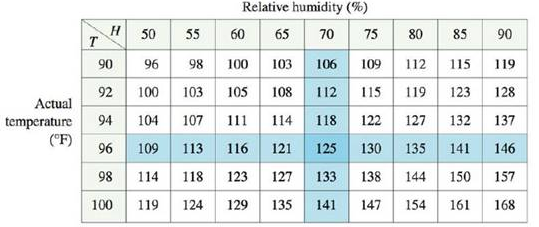Chapter 14.3, Problem 2E

Chapter
Section
Textbook Problem

At the beginning of this section we discussed the function I = f(T, H), where I is the heat index, T is the temperature, and H is the relative humidity. Use Table 1 to estimate fT(92, 60) and fH(92, 60). What are the practical interpretations of these values?Table 1 Heat index I as a function of temperature and humidityTo determine

To estimate: The values of fT(92,60) and fH(92,60) from the Table 1, interpret the values.

Explanation

Given:

The function I=f(T,H) , where I is the heat index, T is the temperature and H is the relative humidity.

Definition used:

“If f is a function of two variables, its partial derivatives are the functions fx and fy defined by

fx(x,y)=limh0f(x+h,y)f(x,y)hfy(x,y)=limh0f(x,y+h)f(x,y)h

Calculation:

Obtain fT(92,60) by definition.

fT(92,60)=limh0f(92+h,60)f(92,60)h

Assume h=2 and obtain the value of fT(92,60) .

fT(92,60)f(92+2,60)f(92,60)2f(94,60)f(92,60)2=1111052=62=3

Similarly, assume h=2 and use Table 1 to obtain the values of fT(92,60) .

fT(92,60)f(922,60)f(92,60)2f(90,60)f(92,60)2=1001052=52=2.5

Obtain the average of above two values of fT(92,60) ,

fT(92,60)=3+522=6+522=1122=114

Thus, the value of fT(92,60)=2

Still sussing out bartleby?

Check out a sample textbook solution.

See a sample solution

The Solution to Your Study Problems

Bartleby provides explanations to thousands of textbook problems written by our experts, many with advanced degrees!

Get Started

A population of N = 15 scores has SX = 120. What is the population mean?

Statistics for The Behavioral Sciences (MindTap Course List)

In problems 1-16, solve each equation. 20.

Mathematical Applications for the Management, Life, and Social Sciences

The graph of is: a) b) c) d)

Study Guide for Stewart's Single Variable Calculus: Early Transcendentals, 8th

True or False: , for –π ≤ t ≤ π, is smooth.

Study Guide for Stewart's Multivariable Calculus, 8th

Find x. log3x=4

College Algebra (MindTap Course List)